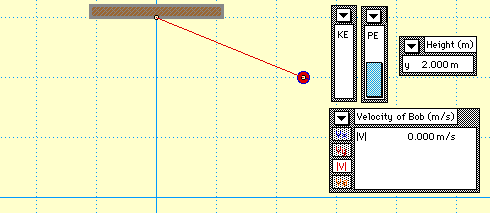Honors Physics‎ > ‎

### How are work and energy related?

posted Jan 27, 2020, 6:11 AM by Barbara Fortunato   [ updated Jan 30, 2020, 4:01 AM ]
 6: F 1/31, 8: F 1/31Today, we'll do a lab where we investigate how work and energy are related.  You'll observe how energy transformations occur in a simple pendulum.  You'll learn to use a Vernier photogate timer to find the velocity of the pendulum.  Here's the setup:Raise the pendulum mass to a height above its lowest (equilibrium) position.  Release and measure the velocity as it passes through its lowest position.  Repeat for at least 6 different release heights.  Graph velocity (m/s) vs. release height (m).  Find the mathematical relationship between velocity and release height, linearizing the graph if necessary.Answer the following analysis questions in your lab notebooks:How much work do you do in raising the the pendulum mass?What forces are on the pendulum as it swings down to equilibrium position?  (Draw a free body diagram.)  How much work does each of the forces do?Write a sentence or two about how mechanical energy is transformed from one form to another.Describe the shape of the velocity vs. height graph.  What does this say about the relationship between velocity and release height. What is the theoretical value of the coefficient in your equation?  How does it compare with your experimentally found coefficient?What assumptions did you make in your experiment?  How would these assumptions affect your coefficient that you found in your mathematical relationship?What is the role of the mass of the pendulum in your equation?  Would a better determination of the value of this mass improve the accuracy or precision of this experiment?Homework:  If you have not finished the analysis for the Pendulum Lab, please finish it for homework.  Lab quiz next Thursday, February 6th.